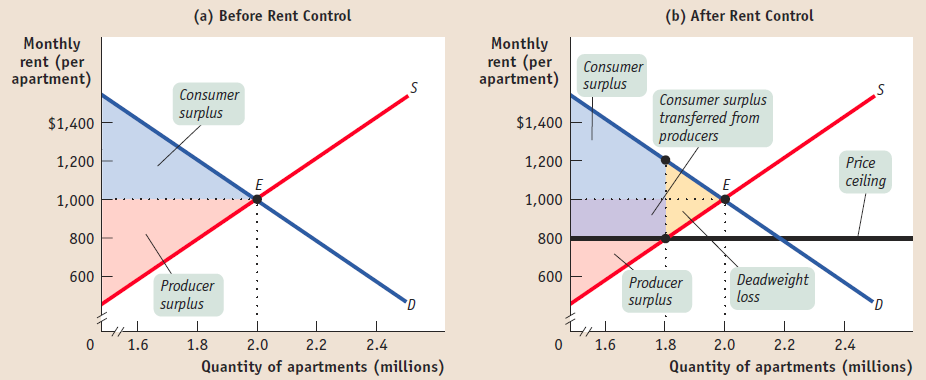# Economics Tutor in Sydney, Melbourne, Perth, and Online in Australia

Best Economics Tutor in Sydney, Perth, MelbourneLoading...Taking too long?Reload document
|Open in new tab

### Market Models

#### Consider two identical firms (A and B), each with a constant marginal cost of 10 and zero fixed costs. The inverse market demand function is: .

1. Derive the Cournot reaction functions for both firms and solve to find the output produced by each
2. Explain what would happen if the two firms now have different
3. Return to the case where each firm has a constant marginal cost of 10 and zero fixed costs. Assuming that firm A is now the Stackelberg leader and firm B is the follower, find the level of output that both firms will now
4. Why there is a difference between the Cournot and Stackelberg equilibrium output levels and prices and what the implications are for the profits made by each firm?
5. If the two firms form a cartel and act as a profit maximizing monopolist, what level of profits would each make?

Does firm 1 have an incentive to deviate from the cartel? If firm 1 deviated from the cartel, state how much the deviation quantities and profits for each firm would be and the market price?

### Game Theory

Consider a simultaneous game in which both players choose once between the actions of ‘Cooperate’ denoted by C and ‘Defect’ denoted by D. Suppose the following payoffs in the game: If both players play C, each gets a payoff of 1; if both play D, both players get 0; and if one player plays C and the other plays D, the cooperating player gets  while the defecting player gets .

1. Illustrate the payoff matrix for this
2. What restrictions on would you have to impose in order for this game to be a Prisoner’s Dilemma?

Now consider a repeated version of this game in which players 1 and 2 meet twice. Suppose you are player 1 in this game and you knew that player 2 would co-operate in round 1 and then copy what you do – known as a ‘Tit-for-Tat’ player.

1. Assuming you do not discount the future, would you ever cooperate with this player?
2. In the repeated game with three encounters, what is the intuitive reason why you might play D in the first stage?
3. Suppose that each time the two players meet, they know they will meet again with probability . Explain intuitively why ‘tit-for-tat’ can be an equilibrium strategy for both players if is relatively large (i.e. close to 1) but not if it is relatively small (i.e. close to 0).

Hire Economics Tutor Sydney for a free trial lesson.

Get Started

## See the #1 economics mentoring platform in action

Request a demo